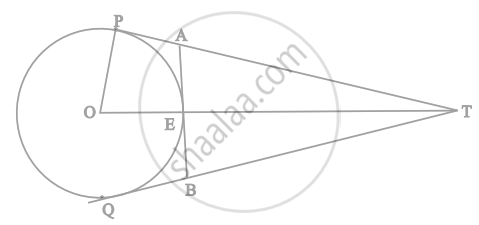# In figure. O is the centre of a circle of radius 5 cm, T is a point such that OT = 13 cm and OT intersects the circle at E. If AB is the tangent to the circle at E, find the length of AB. - Mathematics

Sum

In figure. O is the centre of a circle of radius 5 cm, T is a point such that OT = 13 cm and OT intersects the circle at E. If AB is the tangent to the circle at E, find the length of AB.#### Solution

Given: A circle with center O and radius = 5 cm T is a point, OT = 13 cm. OT intersects the circle at E and AB is the tangent to the circle at E.

To Find: Length of AB

OP ⏊ PT  ......[Tangent at a point on the circle is perpendicular to the radius through point of contact]

By phythagoras theorem in △OPT right angled at P

(OT)2 = (OP)2+ (PT)2

(13)2 = (5)2 + (PT)2

(PT)2= 169 - 25 = 144

PT = 12 cm

PT = TQ = 12 cm  ......[Tangents drawn from an external point to a circle are equal]

Now, OT = OE + ET

ET = OT - OE = 13 - 5 = 8 cm

Now, as Tangents drawn from an external point to a circle are equal.

AE = PA  .....

EB = BQ  ......

Also OE ⏊ AB  ......[Tangent at a point on the circle is perpendicular to the radius through point of contact]

∠AEO = 90°

∠AEO + ∠AET = 180°  .......[By linear Pair]

∠AET = 90°

In ΔAET By Pythagoras Theorem

(AT)2 = (AE)2 + (ET).......[Here AE = PA as tangents drawn from an external point to a circle are equal]

(PT - PA)2 = (PA)2 + (ET)2

(12 - PA)2 = (PA)2 + (8)2  ......[From 1]

144 + (PA)2 - 24PA = (PA)2 + 64

24PA = 80  .......

∠AET + ∠BET = 180  .......[Linear Pair]

90 + ∠BET = 180

∠BET = 90

In ΔBET, By Pythagoras Theorem

(BT)2 = (BE)2+ (ET)2

(TQ - BQ)2 = (BQ)2 + (ET)2  ......[From 2]

(12 - BQ)2 = (BQ)2 + (8)2

144 + (BQ)2 - 24BQ = (BQ)2 + 64

24BQ = 80  ......

So, AB = AE + BE

AB = PA + BQ .......[From 1 and 2]

AB = 10/3 + 10/3   ......[From 3 and 4]

AB = 20/3 cm

Concept: Concept of Circle - Centre, Radius, Diameter, Arc, Sector, Chord, Segment, Semicircle, Circumference, Interior and Exterior, Concentric Circles
Is there an error in this question or solution?

#### APPEARS IN

NCERT Mathematics Exemplar Class 10
Chapter 9 Circles
Exercise 9.4 | Q 11 | Page 111
Share